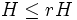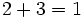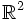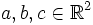# Tour:Examples peek one (beginners)

PREVIOUS: Mind's eye test one (beginners)| UP: Introduction one | NEXT: Inquiry problems one (beginners)
NEXT SECTION Examples peek: Examples peek two
General instructions for the tour | Pedagogical notes for the tour | Pedagogical notes for this part

This page develops some examples of groups in different contexts. The page can be skipped without any loss and content in this page will not be referred to except in future Examples Peek pages. Some of the examples discussed here will be developed from scratch in the main tour, from part four onwards.

The goal of these examples is to provide a hands-on feel to people who think they would benefit from it. Depending on your background, you may have partial familiarity, interest or aptitude for some of these examples. You can concentrate on those examples that suit you best -- the different examples are developed independent of each other.

Another advantage of going through the examples peek is that when you revisit the tour, you'll be able to make sense of more of the content on the pages, particularly the content after the WHAT'S MORE header.

## The group of rational numbers

1. Prove that if$p$ is a prime number, the set of all rational numbers that are either integers or are of the form$a/p^n$ where$a \in \mathbb{Z}$ and$n$ is a natural number, form a subgroup of the group of rational numbers under addition.
2. Prove that if$H$ is a subgroup of$\mathbb{Q}$ and$r$ is a nonzero rational number, then the set of elements of the form$rh, h \in H$ is a subgroup of$\mathbb{Q}$. (here$rh$ denotes the product of rational numbers as numbers, not the group operation, which is addition).
3. Prove that, in the above,$H \le rH$ if$r$ is of the form$1/n$ for some integer$n$.
4. NEEDS SOME THOUGHT: Prove that if$H$ is a proper subgroup of$\mathbb{Q}$ (i.e.,$H$ is not the whole of$\mathbb{Q}$), and$r$ is a nonzero rational number, then$rH$ is also a proper subgroup of$\mathbb{Q}$.
5. NEEDS SOME THOUGHT: Prove that if$H$ is a proper subgroup of$\mathbb{Q}$ (i.e.,$H$ is not the whole of$\mathbb{Q}$) and$H$ is nontrivial in$\mathbb{Q}$, then we can find an integer$n$ such that$H$ is properly contained in$(1/n)H$, which in turn is properly contained in$\mathbb{Q}$.

## Modular arithmetic

Let$n$ be a positive integer. The group of integers modulo$n$ is defined as follows. As a set, it is the set$\{ 0,1,2, \dots, n - 1 \}$. To add two numbers, we first add them as integers. If the sum is again in the set, we declare that as the sum. If the sum is greater than$n$, we subtract$n$ from it.

For instance, the group of integers mod 4 is, as a set,$\{ 0,1,2,3 \}$. Here,$1 + 2 = 3$, whereas$2 + 3 = 1$, because the integer sum is 5 and$5 - 4 = 1$.

1. Prove that the group of integers mod$n$ is a group for every integer$n$, and give explicit expressions for the identity element and inverses in this group. Further, prove that this group is Abelian.
2. Prove that the group of integers mod 1 is the trivial group.
3. In the group of integers mod$n$, find all elements that are equal to their additive inverse. (your answer will depend on$n$).

## Permutations

These are covered in detail in part five of the tour.

For a set$S$, let$\operatorname{Sym}(S)$ denote the set of all bijective maps from$S$ to$S$.

1. Prove that$\operatorname{Sym}(S)$ forms a group where the multiplication is given by function composition.
2. Prove that if$S$ is empty, then$\operatorname{Sym}(S)$ is the trivial group.
3. Prove that if$S$ is a one-point set, then$\operatorname{Sym}(S)$ is the trivial group.
4. Prove that for a set$S$ of size$n$, the size of$\operatorname{Sym}(S)$ is$n!$.
5. Prove that if$S$ has size three, then$\operatorname{Sym}(S)$ is non-abelian.
6. Suppose$S$ is a subset of$T$. Prove that$\operatorname{Sym}(S)$ can be naturally regarded as a subgroup of$\operatorname{Sym}(T)$.

## Vector spaces

This assumes some prior knowledge of vector spaces over fields, or at least, over$\R$:

1. Prove that any real vector space is an abelian group with the usual addition.
2. If$V$ is a real vector space, and$W$ is a vector subspace of$V$, prove that$W$ is a subgroup of$V$.
3. Prove that the zero-dimensional vector space is, as an abelian group, the trivial group.
4. Prove that there are subgroups of a vector space that are not subspaces (Hint: Look at the one-dimensional vector space over the reals).

## Invertible linear transformations

### From a geometric perspective

1. NEEDS LOT OF THOUGHT: Let$\R^2$ denote the (Euclidean) plane. A map$f:\R^2 \to \R^2$ is termed an isometry if, for any two points$p,q \in \R^2$, the distance between$p$ and$q$ is the same as the distance between$f(p)$ and$f(q)$. Prove that any isometry from$\R^2$ to itself is bijective. (Injectivity is not hard; surjectivity requires work; skip the proof of surjectivity if you are unable to get it.)
2. NEEDS SOME THOUGHT: Building on the previous problem, prove that the isometries from$\R^2$ to$\R^2$ form a group under composition.
3. NEEDS SOME THOUGHT: A bijective map$f:\R^2 \to \R^2$ is termed a similarity if the image of any triangle under$f$ is a similar triangle. Prove that the similarities from$\R^2$ to$\R^2$ form a group under composition. (Bijectivity is in fact not necessary as an assumption, and it can be proved in a similar way to problem 1).
4. Prove that the group of isometries is a subgroup of the group of similarities.
5. An invertible collineation is an invertible map$f:\R^2 \to \R^2$ with the property that three points$a,b,c \in \R^2$ are collinear if and only if$f(a), f(b), f(c)$ are collinear. Prove that the invertible collineations form a group under composition.
6. Prove that the group of similarities is a subgroup of the group of invertible collineations.
7. A translation on$\R^2$ is an isometry$f:\R^2 \to \R^2$ with the property that$f$ sends every line to a parallel line. Prove that the translations (under composition) form a subgroup of the group of isometries. (Note: Every line is assumed parallel to itself)

Summarize the findings from all these exercises.

### From a linear algebra perspective

1. Prove that the$n \times n$ matrices with real entries form a group where the group operation is addition. This group is called$M(n,\R)$.
2. Prove that the$n \times n$ matrices with real entries, having nonzero determinant, form a group where the group operation is multiplication. This group is termed$GL(n,\R)$, and is called the general linear group of degree$n$ over$\R$.
3. Prove that the$n \times n$ matrices with all off-diagonal entries equal to zero, form a subgroup of$M(n,\R)$ under addition.
4. Prove that the$n \times n$ matrices with all off-diagonal entries equal to zero, and all diagonal entries nonzero, form a subgroup of$GL(n,\R)$ under multiplication.